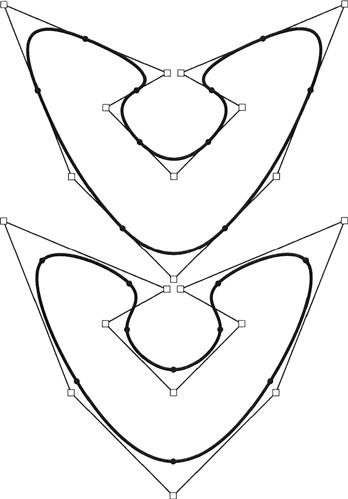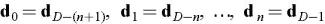The Essentials of CAGD

# 10.7: Periodic B-Spline Curves

## 10.7 Periodic B-Spline Curves

Consider the B-spline curves in Figure 10.11. They show two B-spline curves which are seemingly without beginning or end. Such curves and called periodic.Figure 10.11: Periodic B-spline curves: top, quadratic, bottom: cubic

A periodic B-spline curve can be constructed as a simple special case of a "normal" one. The goal is to have a seamless control polygon, and evaluation at first domain knot and last domain knot produce the same point. Recall that the de Boor algorithm applied to a particular parameter involves only n+1 control points. This gives us the number of control points that must overlap. Taking a closer look at the de Boor algorithm, we can observe that the first n + 1 knot intervals influence the position of the "first" point and the last n+1 intervals influence the position of the "last" point. Thus the conditions are as follows.

Let ? i = u i+1 ? u i. Then the knot sequence should be constructed such that we haveand the de Boor points such thatWe illustrate the principle using a simple cubic example. The curve in the left of Figure 10.12 is not "quite" periodic. It uses a knot sequence without multiple end knots. In this case, the knot sequence is 0, 1, 2, 3, 4, 5, 6, 7, 8. Keep in mind that the curve is only evaluated over the four intervals between [2, 6]. The first control point is the solid...